# Default Algorithm

This algorithm is used to generate a module file using basic datasheet inputs and default assumptions. At its core is the single diode equivalent circuit model for photovoltaic modules (as defined by the circuit diagrams below for modules with and without recombination; the recombination current path is shown as a dotted line).The equation governing this equivalent circuit is achieved using Kirchoff’s current law, which states that current must sum to zero at any junction of a circuit: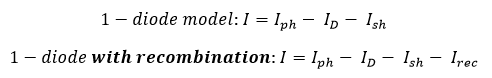The resulting governing equations are:and all other variables are defined below in “Inputs”. The particular application of the single diode model is based on a process and set of assumptions developed by Andre Mermoud. All of the required inputs can be sourced from a standard module datasheet from any manufacturer.1. Calculate shunt resistance and dark shunt resistance.

The default shunt resistance is calculated according to the following equation quantized (rounded) to a step size dependent on what range the raw calculated value lies in.…where K is the “shunt resistance multiplier”, a technology specific multiplier (defaulted to 5.0 for c-Si modules and 3.0 for CdTe modules). The table below specifies the quantization (rounding interval) for each range of raw shunt resistance values.Rsh,dark resistance is calculated by multiplying Rsh by a constant and quantized similarly to shunt resistance.where Kdark is the dark shunt multiplier. The table below specifies the quantization (rounding interval) for each range of raw  values.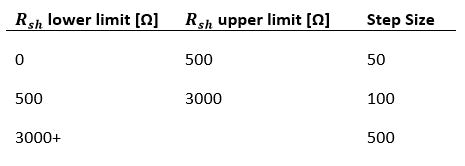2. Calculate the recombination parameter if the Model Type is “1-Diode with Recombination” (skip otherwise).

If generating a module with recombination, the recombination parameter must be estimated, which is derived from the maximum recombination parameter. In order to find the maximum recombination parameter, series resistance is set to (approximately) zero. The algorithm then increments recombination parameter, starting at zero, and solves a system of equations at each iteration.

The system of equations is defined using the “1-diode with recombination” governing equation above at 3 different conditions: (I = Isc, V = 0), (I = 0, V = Voc), and (I = Imp, V = Vmp). The Levenberg-Marquadt non-linear least squares solving algorithm is used to solve the system of equations and calculate the following unknowns: Iph (light generated current), γ, and I0. The initial guesses for solving the system of equations are as follows:The iterative loop ends when the condition I0/Iph < 1 x 10-12 is met. The value of recombination parameter (di2/μτ) from the previous iteration before this limit is reached is taken to be the maximum recombination parameter. The final value for the recombination parameter is calculated using the simple relationship:3. Calculate maximum series resistance.

The next step is to calculate the maximum theoretical series resistance to serve as an upper limit for calculating the actual series resistance. The algorithm iterates through incrementally increasing values of Rs, starting from (nearly) zero. Upon each iteration, a system of equations is solved to determine the optimal values for unknowns, similarly to step 2. The appropriate governing equation (“1-diode model” or “1-diode with recombination”) should be used. Additionally, for the non-recombination “1-diode model” use case, the only unknowns calculated from the system of equations are I0 and γ. Light-generated current is calculated with the following equation:where Rs,i is the ith iteration’s series resistance value. The iterative loop ends when either no solution can be found, or the ratio I0/Iph < 1 x 10-12. This ratio is a somewhat arbitrarily, but empirically-determined limit. The value of Rs in the previous iteration before this limit is reached is Rs,max.

4. Calculate series resistance and final single diode parameters.

1-diode model (no recombination)

For the “1-diode model” use case, determine the series resistance value that will yield 97% relative efficiency at 200 W/m2 and 25⁰C. The algorithm does this by iterating through values of Rs between 20% and 95% of Rs.max (determined in the previous step). Upon each iteration, the system of equations is solved similarly to previous steps using the “1-diode model” governing equation and the same three conditions. The solution to the system of equations is used to calculate of the linear dependence of γ (µγ) (see separate documentation for the µγ calculation). The relative efficiency at 200 W/m2 is then calculated (see separate documentation for the EIR algorithm).

At the end of each iteration, error between the calculated EIR value at 200 W/m2 and the desired value of 0.97 is recorded. The error calculated in the current iteration is compared to the previous iteration. If the sign of the error changes (i.e. positive to negative error), then the value of Rs from the previous iteration is chosen as optimal.

The values for I0, γ, and μγ calculated in the iteration corresponding to the optimal Rs value are the final single diode parameters.

1-diode Model with Recombination

In the case of recombination, series resistance is calculated using the simple relationship:The values for I0 and γ by solving the appropriate system of equations with the final value of series resistance, and used as inputs for calculating μγ.

# Linear Temperature Dependence of Diode Ideality Factor Calculation

This algorithm calculates the linear temperature dependence of the diode ideality factor. In general, it does this by determining a value that results in a temperature coefficient of maximum power as close as possible to the datasheet input.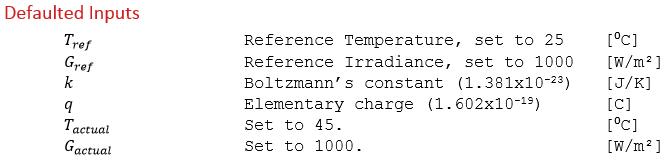1. Determine the direction that mu gamma should increment.

The linear temperature dependence of gamma is incremented once in both the positive and negative directions, creating a set of three values:For each of these values, γ is temperature adjusted to 45⁰C, in addition to temperature adjusting all of the other single diode parameter inputs (see the algorithm documentation section entitled “One-Diode Model Temperature Correction”).

Calculate Pmp at STC then for each of the three mu gamma values, calculate Pmp at 45⁰C and calculate mu_Pmp using the following equation:If the error between calculated mu_Pmp and mu_Pmp from the datasheet input decreases for mu_gamma + increment, then mu_gamma should be incremented in the positive direction to achieve the optimal value.

1. Iterative increment to the optimal value that generates close to the inputted value of mu_Pmp.

Increment mu_gamma in the direction determined in step 1, performing a similar calculation to step 1 and checking the error between calculated_mu_Pmp and mu_Pmp at each iteration. The optimal value of mu_Pmp that which precedes the error between calculated_mu_Pmp and mu_Pmp starting to increase.

# Effective Irradiance Response (EIR) Calculation

The effective irradiance response (EIR) of a module is its relative efficiency at various non-standard conditions. This is sometimes referred to as a module’s “low-light response”. The following algorithm describes how to calculate the relative efficiency at a single condition (non-STC temperature/irradiance). This process can be repeated at various conditions to generate an EIR curve.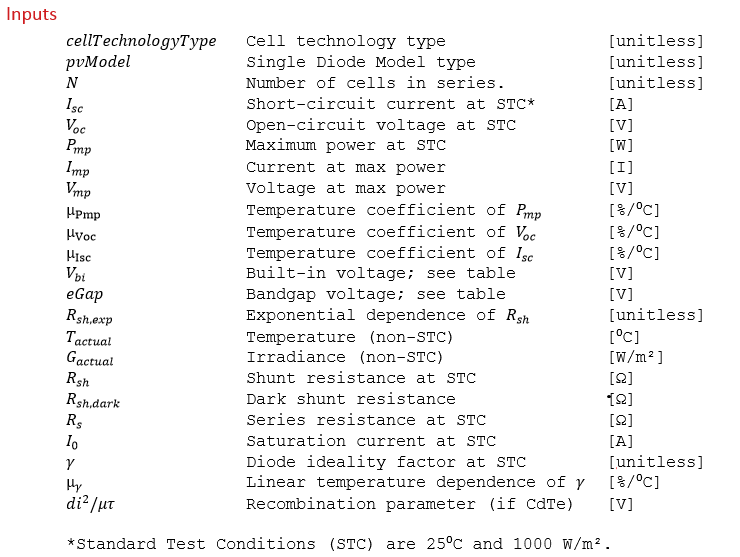1. Calculate the maximum power point at Standard Test Conditions (STC).

The maximum power point is calculated using single diode parameters at STC.

1. Temperature and irradiance adjust all pertinent single diode parameters to T_actual and G_actual.

See the algorithm documentation section entitled “One-Diode Model Temperature Correction”.

1. Calculate the maximum power point from the temperature/irradiance-adjusted single diode parameters.

Using the temperature-adjusted single diode parameters, calculate the maximum power point as in step 1.

1. Calculate the relative efficiency.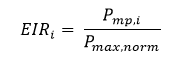where EIRi is the relative efficiency at a given irradiance in decimal form,  Pmp,i is the maximum power point at a given irradiance, and Pmax,norm is the normalized maximum (ideal) power point at a given irradiance, as described by the following equation:where Pmp,1000 is the maximum power point at 1000 W/m2 and i is the given irradiance.

# “Optimize Series Resistance to Match EIR” Algorithm

This algorithm finds the optimal series resistance value (with all other single-diode parameters constant) that generates a model-calculated effective irradiance response (EIR) close to some target (user-specified) EIR. In this way, the algorithm “tunes” a module’s series resistance to a desired EIR.1. Determine the direction that series resistance should increment.

Starting from Rs,max/2, series resistance is incremented once in both the positive and negative directions. The relative efficiencies at 800, 600, 400, and 200 W/m2 are calculated for each of the three series resistances: Rs,max/2 – increment, Rs,max/2, Rs,max/2 + increment (see “Module File Generation: Effective Irradiance Response (EIR) Calculation”). The error between each of the three generated EIR’s and the target EIR is calculated using a weighted RMS error:where, for example,If adding an increment to Rs,max/2 decreased the error, then the algorithm will increment in the positive direction (and vice versa).

1. Iteratively find the optimal value of series resistance that generates the closest EIR to the target EIR.

The algorithm then increments Rs in the direction determined in step 1, calculating EIR error at the end of each iteration using the equation in step 1. It does this until the EIR error begins to increase again, Rs reaches Rs,max (in the case that the increment is positive), or Rs reaches 0.05Rs,max (in the case that the increment is negative). Using that final value of Rs, the following single diode parameters are recalculated: I0, γ, and μγ.

# Processing Key I-V Points

The “Key I-V Points” input option provides an alternative to “basic datasheet” parameters as input for the module file generating algorithm. This is a pre-processing step to the core module file generator. This input format is modeled after the IEC-61853-1 test standard, defined by the International Electrotechnical Commission (IEC) as: “requirements for evaluating PV module performance in terms of power (watts) rating over a range of irradiances and temperatures…a second purpose is to provide a full set of characterization parameters for the module under various values of irradiance and temperature”. See the “Inputs” below for more information.*Important Note: These inputs are intended to be provided for multiple temperature/irradiance conditions (, ).Each set of inputs is represented by a row in the Key I-V Points user interface. The minimum required input is data at STC.Key IV Points Algorithm

1. The only required row of inputs is for STC conditions. The provided values for maximum power, voltage at maximum power, current at maximum power, short-circuit current, and open-circuit voltage are assumed to be the final “Electrical Characteristics at STC” of the module.

1. If data is provided at multiple temperatures, temperature coefficients will be calculated for Pmp, Voc, and Isc. This is done by performing a linear regression where the x-values are temperature, and the y-values are the respective electrical characteristic at each temperature (and G = 1000 W/m2). The resulting temperature coefficients are calculated in units [%] by dividing the slope of the linear fit by the respective electrical characteristic at STC.

1. For each temperature, if data is provided at multiple irradiances, relative efficiency at each irradiance is calculated from each as in step 4 of the documentation for “Effective Irradiance Response (EIR) Calculation”.

# Processing Full I-V Curves

The “Full I-V Curves” input option provides another alternative “basic datasheet” parameters as input for the module file generating algorithm. This is a pre-processing step to the core module file generator.*Important Note: I-V curves intended to be provided for multiple temperature/irradiance conditions (, ). The minimum required input is data at STC.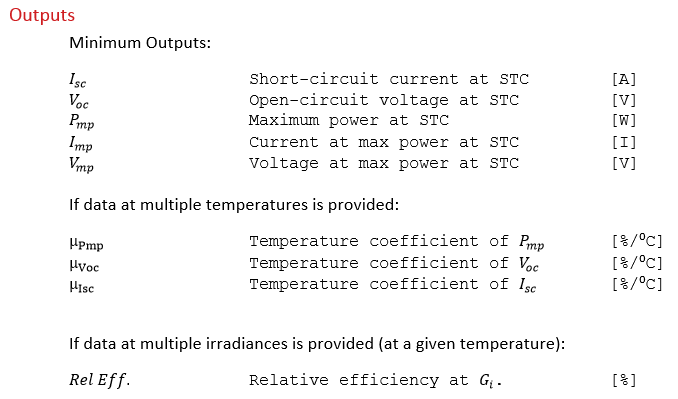Full IV Curves Algorithm

For each I-V curve (at a unique set of temperature and irradiance conditions):

1. Short-circuit current is extracted by taking the value of current where voltage is equal to zero. If the I-V curve does not include a point at the I-intecept, the closest point is taken and an interpolation is made to the I-axis using the slope of the I-V curve nearest to the I-axis.

1. Open-circuit voltage is extracted by taking the value of voltage where current is equal to zero. If the I-V curve does not include a point at the V-intecept, the closest point is taken and an interpolation is made to the V-axis using the slope of the I-V curve nearest to the V-axis.

1. The maximum power point is determined by multiplying every set of current and voltage values together, and taking the maximum of that resulting list. The current at maximum power and voltage at maximum power are those that resulted in the maximum power point.

After the parameter extraction is performed on each provided I-V curve, the resulting data is processed as described in the documentation for “Key I-V Points” to calculate temperature coefficients and relative efficiencies.